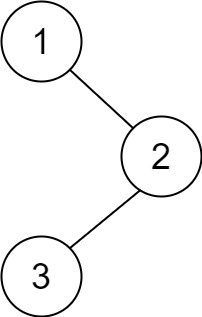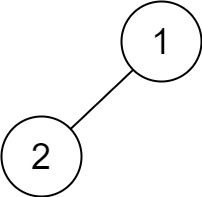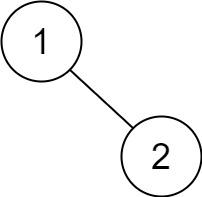# LeetCode | 145. Binary Tree Postorder Traversal

Given the root of a binary tree, return the postorder traversal of its nodes' values.

Example 1:Input: root = [1,null,2,3]
Output: [3,2,1]


Example 2:

Input: root = []
Output: []


Example 3:

Input: root = 
Output: 


Example 4:Input: root = [1,2]
Output: [2,1]


Example 5:Input: root = [1,null,2]
Output: [2,1]


Constraints:

• The number of the nodes in the tree is in the range [0, 100].
• -100 <= Node.val <= 100

Recursive solution is trivial, could you do it iteratively?

/**
* Definition for a binary tree node.
* struct TreeNode {
*     int val;
*     TreeNode *left;
*     TreeNode *right;
*     TreeNode(int x) : val(x), left(NULL), right(NULL) {}
* };
*/
class Solution {
public:
void backPostorder(TreeNode* root, vector<int>& res) {
if(root == NULL)
return;
backPostorder(root->left, res);
backPostorder(root->right, res);
res.push_back(root->val);
return;
}
vector<int> postorderTraversal(TreeNode* root) {
vector<int> res;
if(root == NULL)
return res;
backPostorder(root, res);
return res;
}
};

01-164189
08-2044509-17254
05-26838
05-256613
08-16596
05-231274
06-021073
01-29160
05-23159